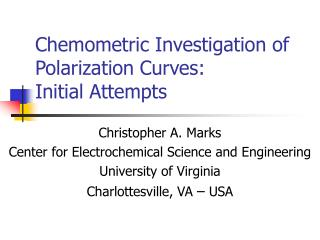# Chemometric Investigation of Polarization Curves: Initial Attempts - PowerPoint PPT PresentationDownload PresentationChemometric Investigation of Polarization Curves: Initial Attempts

Chemometric Investigation of Polarization Curves: Initial AttemptsDownload Presentation## Chemometric Investigation of Polarization Curves: Initial Attempts

- - - - - - - - - - - - - - - - - - - - - - - - - - - E N D - - - - - - - - - - - - - - - - - - - - - - - - - - -
##### Presentation Transcript

1. Chemometric Investigation of Polarization Curves:Initial Attempts Christopher A. Marks Center for Electrochemical Science and Engineering University of Virginia Charlottesville, VA – USA

2. Outline • Polarization Curves • Definition and Terms • Motivation for Chemometric Approach • Differences from Spectroscopic Data • Data • Methods • Results • Future Work • Acknowledgements

3. Polarization Curves Electrochemical measurements of net current density (i (A cm-2)) as a function of potential (E (V)) for a given electrolyte and working electrode

4. Polarization Curves – H2O Reactions

5. Polarization Curves – Metal Reactions

6. Polarization Curves – Net Current Measurement • inet = ianodic + icathodic • M  Mn+ + ne- • 2H20  O2 + 4H+ + 4e- • O2 + 4H+ + 4e-  2H2O • Only a small fraction of what is of interest can be measured experimentally

7. Polarization Curves – Motivation Resolve net current data into components which are simple functions of pH, [Cl-], [O2], [Mn+], etc. so that the important parameters, Eoc, Ep, and ipass, can be optimized or interpolated.

8. Polarization Curves – Different from Spectra • Non-constant domain (non-random missing data) • Variable uncertainty in i, depends on i, not E • How to calculate 2?

9. Polarization Data • ~ 2 alloys • 3 temperatures • 2 electrolytes • ~ 3 pHs • 240 (partial) polarization curves – 8 shown

10. Methods • PCA-like approach in Matlab (NIPALS) • No mean-centering or scaling • Missing values replaced by estimates (Xestij=tipj) • Iteratively re-weighted least squares • Estimate loadings (p=(t’t)-1t’X) • Calculate variable weights (v) based on p(vi = # obs / (a priori uncertainty for pi)2 • Estimate scores (t=Xdiag(v)p’(pdiag(v)p’)-1) • Go to 1, until convergence • Orthogonalize p with respect to the previous P

11. Results • First factor appears fine, others are less compelling • Several outliers identified and removed • Algorithm is slow to converge

12. Results

13. Results

14. Future Work • PCA • Verify target function and weighting • Non-orthogonal P • Simulated data • Smaller/simpler data sets • Non-negative T (P?) and/or Rotations • PLS and other techniques • Time series • EIS, 3-way? • Spatial electrode arrays

15. Acknowledgements Beth Kehler – UVa MS (2001), now at Intel B.A. Kehler, G.O. Ilevbare, J.R. Scully, "Comparison of the Crevice Corrosion Resistance of Alloys 625 and 22," CORROSION/2000, paper no. 182, NACE, 2000. B.A. Kehler, Crevice Corrosion Electrochemistry of Alloys 625 And C22, University of Virginia, Charlottesville, January, 2001. John Scully, Rob Kelly, et al. – CESE Jack McArdle – UVa Psychology WSC1 presenters and participants